WEBINAR
Efficient Green Energy: Exploring Simulation-Driven Design for High-Torque Wind Turbine Machine
Thursday, September 21, 2023
Time
SESSION 1
SESSION 2
CEST (GMT +2)
03:00 PM
08:00 PM
EDT (GMT -4)
09:00 AM
02:00 PM
HOME / Applications / Synchronous Generator Performance Evaluation Under Different Loads Using EMWorks 2D

# Synchronous Generator Performance Evaluation Under Different Loads Using EMWorks 2D

Used Tools:## Introduction

Synchronous generators are the most popular energy converter to generate electrical energy from mechanical energy . Although they are widely used at different power stations, they can also be found in transportation applications such as aircraft and ships. In this application note, a typical synchronous generator used in an aircraft electrical system is studied under different load conditions. Its performance is investigated using the finite element method (FEM) tool in EMWorks 2D software. The circuit coupling feature available in the software package is used to analyze the synchronous generator with three different types of load such as resistive, inductive, and capacitive load. For comparative analysis, the magnitude of the load is kept constant and the load angle is varied. The output parameters of the machine such as load current, back EMF, and terminal voltage are compared.

## Case Study

The machine used in this study is a salient pole synchronous generator, equipped with 10 rotor poles and 30 stator slots as shown in Figure 1. The stator slot is wounded in a double-layer winding configuration having a winding factor of 0.866. The generator parameters are as shown in Table I. In general, the machines used in the aircraft application are smaller in size and are designed for higher operating frequency. Hence the base speed of the machine considered under study is set at 12000 rpm.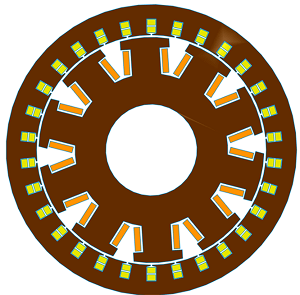Figure 1. A 2-D Cross-Section View of the Synchronous Generator Under Study

Table I: Synchronous Machine Parameters
 Mechanical Speed (rpm) 12000 Rated Power (kVA) 200 Output Voltage (V) 115 Rated Current (A) 577 Rotor Excitation DC Current (A) 180 Stator and Rotor Core Material M27

## Comparative Analysis and Results

In this section, the synchronous generator under three different load conditions is simulated. In all cases, the impedance magnitude of the load is kept equal to 10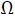, while the load angle is varied to achieve the power factor of 1 (unity), 0.65 leading and 0.65 lagging. A balanced three-phase load with a star connection is considered for all the load conditions and the simulation is performed using the EMWorks 2D software. The DC excitation of the rotor is equal to 180 A and the rotor mechanical speed is equal to 12000 rpm for all the conditions. Based on the following equations, the resistance, inductance, and capacitance values are calculated and used in the simulations. Table II summarizes the different load types and the corresponding inputs of each study. Figure 2 shows one example of the synchronous generator connected at 0.65 lagging load.

Figure 3 shows the magnetic flux density plot of the synchronous generator connected to a purely resistive load at t = 0.5 ms. As observed from the plot, the rotor poles get saturated at this instance and the magnitude of magnetic flux density is approximately equal to 2.12 T. A similar flux distribution plot can be obtained for the other load conditions.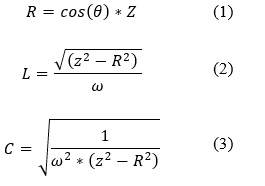Case Load Power Factor R () L (mH) C (mF) Load Angle
1 1 (unity) 10 0 0
2 0.65 lagging 6.43 1.22 0 50°
3 0.65 lagging 6.43 0 0.02078 -50°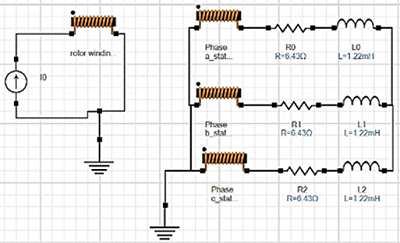Figure 2. Modelled Circuit Schematic of the Synchronous Generator at 0.65 Lagging Load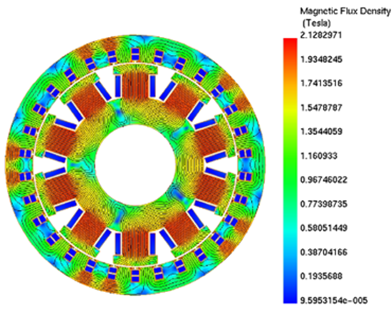Figure 3. Magnetic Flux Density Distribution of a Synchronous Generator at Purely Resistive Load

In this section current and voltage, waveforms are plotted vs. time for each case. It can be observed that the currents are shifted from voltage due to the difference in the load angle. Figure 4 represents case 1 results with a purely resistive load, it can be observed that the current and voltage are in phase with each other. Figure 5 represents case 2 results with RL load, here voltage leads with the current. Similarly, Figure 6 represents case 3 results with RC load where voltage lags with the current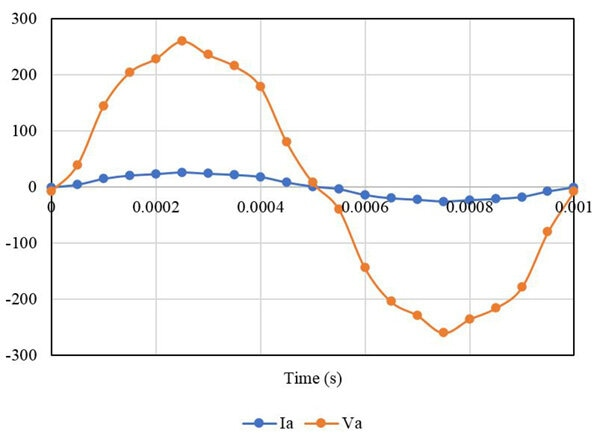Figure 4. Output Current and Voltage Waveforms with the Purely Resistive Load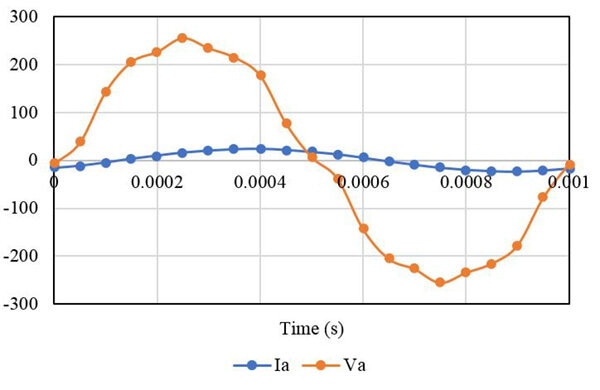Figure 5. Output Current and Voltage Waveforms with the RL Load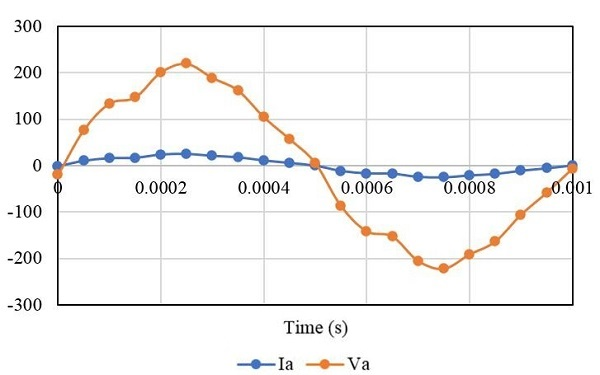Figure 6. Output Current and Voltage Waveforms with the RC Load

Table III: Output Current and Voltage Amplitudes Comparison for Different Types of Load
Current Voltage Current Voltage
R 25.97 A 259.7 V 18 A 180 V
RL 24 A 255.3 V 16.6 V 178.6 V
RC 25 A 219.5 V 16.7 A 146.8 V

Referring to Table III, it can be inferred that the maximum current is obtained by a purely resistive load whereas the minimum current is performed by the RL load. Moreover, the difference between the magnitude of the output current obtained among all the cases is small as their impedance magnitude is equal.

Figure 7 represents the output three-phase back EMF waveforms of the synchronous generator. The back EMF voltage is obtained by supplying the rotor excitation current and the armature winding circuit is kept open as shown in Figure 8. The back EMF waveform remains the same for the three loads. Fig 9,10 and 11 present the back EMF produced by the generator at different speeds, excitation currents, and the number of turns of the rotor winding. Fig 9 confirms that the generated power has a linear behavior regarding the speed.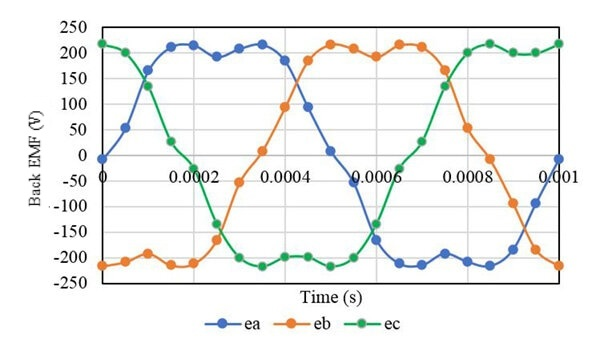Figure 7. Back EMF Waveforms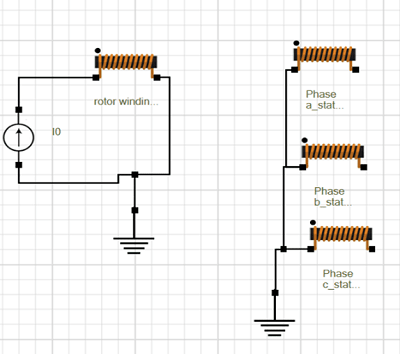Figure 8. Open Circuit Schematic for Back EMF Simulation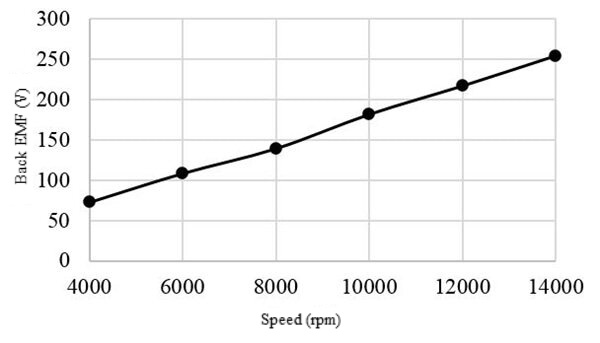Figure 9. Back EMF vs. Speed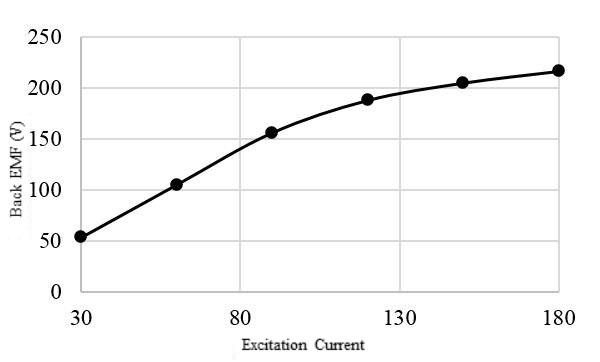Figure 10. Back EMF vs. Excitation Current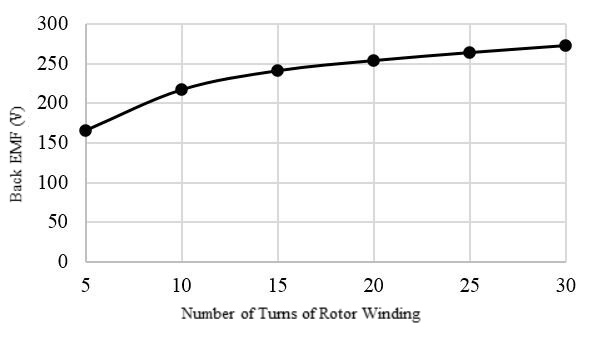Figure 11. Back EMF vs. the Number of Turns of the Rotor Winding

## Conclusions

In this application note, three different types of load such as pure resistive, RL and RC load keeping the same impedance are compared for synchronous generators. The electromagnetic performances such as output current, output voltage, and the back EMF are obtained using the EMWORKS 2D software. It has been found that the load angle affects the phase delay between the output current and voltage waveform. Also, the back EMF at different speeds and excitation current values are simulated and analyzed.

#### References

 Wildi, Theodore. Electrical Machines, Drives and Power Systems. 6th ed. Upper Saddle River: Pearson Practice Hall, 2006. 377-396 (Wildi, 2006)